# Circles

The concepts of circles are crucial for the CAT quantitative aptitude section because several direct and indirect questions are asked from this. Also, many geometry questions require the concept of circles to be able to solve them. Learn the concepts of circles, important terms and definitions and various properties to prepare for the CAT effectively.

## Definition of Circle:

A circle is a closed curve formed by a set of points on a plane and those points are in the same distance from its center O. That distance is called as the radius of the circle.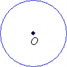## Terms related to a Circle:

### a) Diameter

The diameter of a circle can be defined as a line segment that passes through the center of the circle and has its endpoints on the circle. All the diameters of the same circle have the same length.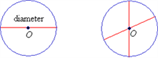A line segment from the center of the circle to a point on the circle is called as the radius of a circle.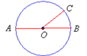In the above diagram, O is the center of the circle and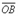and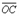are radii of the circle. The radii of a circle are all the same length. The radius is half the length of the diameter.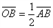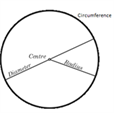### c) Semi-circle

Half of a circle cut off by a diameter is called a semi-circle.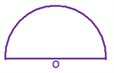### d)Arc

An arc is just a part of a circle. An arc can be measured in degrees.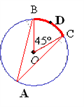In the above diagram, the part of the circle from B to C forms an arc.Also, arc BC is equal to the BOC that is 45°.

### Important Terms of Arcs:

(i) Measure of an Arc

1) The measure of a semi-circle is 1800

2) The measure of a minor arc is the measure of its central angle. In the figure, BC is the minor arc with the measure 45>sup>0

3) The measure of a major arc is 3600-(measure of corresponding minor arc) m(arc BAC)=3600-m(Arc BDC) = 360- 45= 3150

(ii) Intercepted Angle

An angle with vertex (A) as a point of the arc other than its end-points(B&C) and each side of the angle containing one endpoint (B&C) of the arc is called the angle inscribed in the arc. CAB is inscribed in the arc BAC.

(iii) Intercepted Arc

An arc is said to be intercepted by an angle if each side of the angle contains an endpoint of the arc, and the arc but for its endpoints, lies in the interior of the angle. Arc BD and arc AC are intercepted by the AOC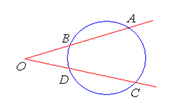### e) Tangent

A tangent is a line that touches a circle at only one point. A tangent is perpendicular to the radius at the point of contact.In the above diagram, the line containing the points B and C is a tangent to the circle. It touches the circle at point B and is perpendicular to the radius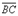is perpendicular toi.e.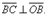### f) Chord

A chord is also a line segment with both endpoints on the circle, but it may not pass through the center of the circle.

### g) Secant

It is a line which intersects the circle in two distinct points.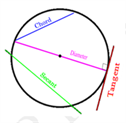### h) Circumference

The circumference of a circle is the distance around a circle.

The formula for the circumference of a circle is C = pd (p (pi) =3.142)

or

C = 2pr

where C is the circumference, d is the diameter and r is the radius.

### i) Area

The area of a circle is the region enclosed by the circle.

It is given by the formula:

A = pr2 where A is the area and r is the radius.

## Types of circles:

### (i) Concentric Circles:

Circles lying in the same plane with a common center are called concentric circles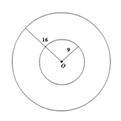### (ii) Tangent Circles:

Circles lying in the same plane and having only one point in common are called tangent circles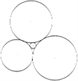One and only one circle passes through three given non-collinear points An infinite number of circles pass through two given points.

### Important Properties Of Chords, Tangents and Secants:

#### PROPERTY 1

The perpendicular from the center of a circle to a chord of the circle bisects the chord. Conversely, the line joining the center of the circle and the midpoint of the chord is perpendicular to the chord.

If OM =AB, then AM=MB and If AM=MB., then OM=AB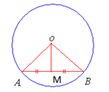#### PROPERTY 2

Equal chords of a circle or congruent circles are equidistant from the center Conversely, two chords of a circle or of congruent circles that are equidistant from the center and are making the same angle with the line drawn from the centre of the circle are equal.

If AB=CD, then OM=ON and if OM=ON, then AB=CD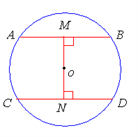#### PROPERTY 3

In a circle or congruent circles, equal chords subtend equal angles at the centre Conversely, chords which subtend equal angles at the center of the same or congruent circles, are equal

If AM=CD, then m ?COD=m ?AOB and If m ?COD= m ?AOB, then AB=CD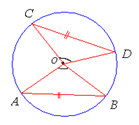#### PROPERTY 4

Tangent Perpendicularity Theorem:

The tangent at any point of a circle and the radius through that point are perpendicular to each other. If O is the center of the circle, A is the point of contact of the tangent X, then OA ? X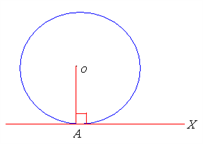Given a point on a circle, there is one and only one tangent to the circle passing through that point

From an exterior point of the circle, exactly two tangents can be drawn onto the circle.

#### PROPERTY 5

The lengths of two tangent segments, from the exterior point to the circle, are equal. AC=BC.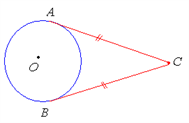This can go on to form a kite as follows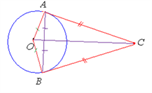5.1) The point of contact of two tangent circles lies on the straight line through the centres.

5.2) If the two circles touch externally, distance between their centres= sum of their radii.

Distance between centres= AC+BC

5.3) If the two circles touch internally, distance between their centers=difference of the radii. Distance between centres= AC-BC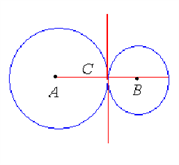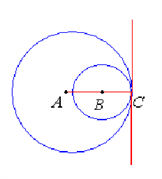#### PROPERTY 6

Angle subtended by a diameter

(i) A diameter subtends an obtuse angle at a point in the interior of the circle m ?AEB>900

(ii) A diameter of a circle subtends an acute angle at a point in the exterior of the circle .m ?ACB<900

(iii) A diameter of a circle subtends a right angle at a point on the circle. m ?ADB=900

Conversely, if a segment subtends a right angle at a point on the circle, then the segment is the diameter of the circle

#### PROPERTY 7

Angles inscribed in the same arc are equal m ?AOB=m ?ACB as they are inscribed in the same arc ADB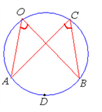#### PROPERTY 8

Equal arcs of a circle or congruent circles have equal chords Conversely, equal chords of a circle or congruent circles have their corresponding arcs equal

If m(arc AXB)=m(arc CYD), then AB=CD and If AB=CD, then m(arc AXB)= m(arc CYD)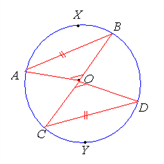#### PROPERTY 9

Inscribed angle theorem:-The measure of an inscribed angle is half the measure of its intercepted arc. m ?ACB= 1/2m(arc ADB)=1/2 m ?AOB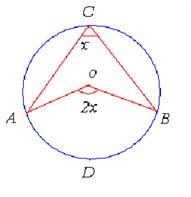#### PROPERTY 10

The angle that the tangent to the circle makes with a chord drawn from a point of contact is equal to the angle subtended by that chord in the alternate segment of the circle

In the following figure, x1=x2=x3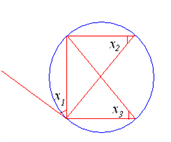#### PROPERTY 11

In the following figure, a+b=1800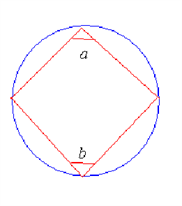#### PROPERTY 12

If a line segment joining two points subtends equal angles at two other points lying on the

same side of the line containing the segment, then the four points lie on the same circle.

Points A,B,C,D lie on the same circle; i.e. they are concyclic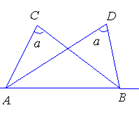#### PROPERTY 13

If two secants intersect in the exterior of the circle, the angle so formed is equal to half the difference of the measures of the arcs intercepted by them.

m(AOC)=1/2[m(arc AC)-m(arc BD)]

Also, AO x BO = CO x DO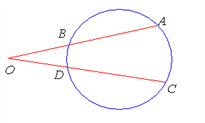#### PROPERTY 14

If two secants intersect in the interior of the circle, the angle so formed is equal to half the sum of the measures of the arcs intercepted by them.

AOC=1/2[m(arc AC)+m(arc BD)] Also, AO x BO = CO x DO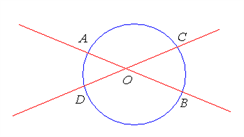Note:- O need not be the origin

#### PROPERTY 15

If a tangent and a secant intersect in the exterior of the circle, the angle so formed is equal to half the difference of the measure of the arc intercepted by them m ?AOC=1/2[m(arc AC)-m(arc BD)] Also AO X BO = CO2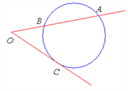#### PROPERTY 16

Common Tangents

16.1) For the two circles with centres A and B, PQ and RS are the direct common tangents and CD and EF are the transverse common tangents (Only two of both direct common and transverse common tangents are possible).

Where r1 and r2 are the radii of the two circles Length of Direct common tangent = v((Distance between centres)2 – (r1-r2 )2 )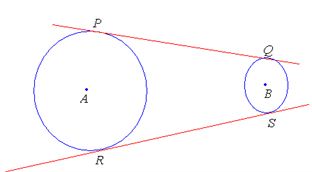Length of transverse common tangent = v((Distance between centres)2 – (r1+r2 )2 )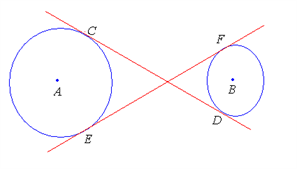To learn more important topics in a similar way, stay tuned with BYJU’S. Also, get various CAT sample papers, preparation tips and engaging video lessons to prepare for the CAT exam more effectively.